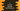# C program to convert seconds into hour/minute/seconds### C program to convert seconds into hour minute and seconds :

In this tutorial, we will learn how to convert a seconds value to hours,minutes and seconds. User will enter the seconds value and our program will divide it in hours, minutes and seconds. For example, 3600 means 1 hour,0 minute and 0 seconds. Let’s take a look at the program :

### C program :

``````#include<stdio.h>

int main(){
//1
int inputSecond;

//2
int hours,minutes,seconds;
int remainingSeconds;

//3
int secondsInHour = 60 * 60;
int secondsInMinute = 60;

//4
printf("Enter seconds : ");
scanf("%d",&inputSecond);

//5
hours = (inputSecond/secondsInHour);

//6
remainingSeconds = inputSecond - (hours * secondsInHour);
minutes = remainingSeconds/secondsInMinute;

//7
remainingSeconds = remainingSeconds - (minutes*secondsInMinute);
seconds = remainingSeconds;

//8
printf("%d hour, %d minutes and %d seconds",hours,minutes,seconds);
}``````

### Explanation :

The commented numbers in the above program denote the step number below :

1. Create one integer variable to store the user-input seconds.
2. Create three integer variables hours,minutes and seconds to store the final hour , minutes and seconds value after splitting the user input value. Integer remainingSeconds is used to temprarily hold remaining seconds value below.
3. secondsInHour denote the total number of seconds in one hour i.e. 3600 or 60 * 60. secondsInMinute denote the total seconds in one minute.
4. Ask the user to enter total seconds. Read and store it in variable inputSecond.
5. Find the hours by dividing the user input seconds by total seconds in one hour.
6. Calculate the remaining seconds by subtracting total seconds in the calculated hour from the total user given seconds. Using these remaining seconds, calculate the minutes by dividing it seconds in one minute.
7. Again calculate current remaining seconds. These remaining seconds are required value for seconds.
8. Print out all hours , minutes and seconds values.

### Sample Output :

``````Enter seconds : 3600
1 hour, 0 minutes and 0 seconds

Enter seconds : 2345
0 hour, 39 minutes and 5 seconds

Enter seconds : 60
0 hour, 1 minutes and 0 seconds``````

You might also like :